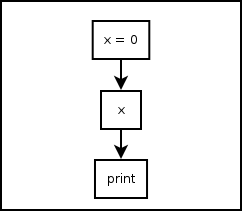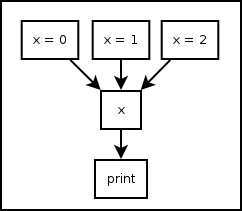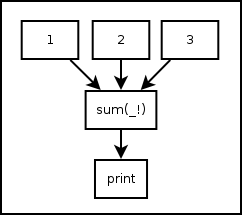# 4. Variables¶

## 4.1. Assignment Statement¶

Pythonect supports standard Python types, but with a twist!

### 4.1.1. Variable <slug> in Single Thread¶

You can define a variable and set its value as follows:

`[x = 0] -> x -> print`

Graphically (Visual programming wise), this is represented as:Both versions print 0. You can also change its value during the program runtime as follows:

`[x = 0] -> x -> print -> [x = 1] -> x -> print`

This will print 0 and 1, in this order, and in the same thread. Of course you can assign and re-assign any legal Python value, for example:

`[x = 0] -> x -> print -> [x = "Hello, world"] -> x -> print`

Will print 0, and "Hello, world"

### 4.1.2. Variable <slug> in Multithreading¶

You can define a variable and set it with multiple values at the same time (each value map()‘ed to a different thread) as follows:

`[x = 0, x = 1, x = 2] -> x -> print`

Graphically (Visual programming wise), this is represented as:Both versions will print, each in its own thread, and not necessarily in that order: 0, 1, 2.

Of course you can assign any combinations of Python value and types, for example:

`[x = 1, x = 1, x = 0.34, x = "Hello, world"] -> x -> print`

This will print, each in its own thread, and not necessarily in that order: 1, 1, 0.34, and "Hello world".

## 4.2. Predefined Variables¶

Pythonect predefines two variables: _ and _!

### 4.2.1. _ as Current Value¶

The variable underscore (i.e. _) is predefined to be the current value on the flow, for example:

`1 -> _ + 1 -> print`

Will print 2. As _ will be equal 1 after evaluating the 1 expression. Another example is:

`["To be", "Not to be"] -> print "To be, or not to be? " + _`

This will print, each in its own thread, and not necessarily in that order: To be, or not to be? To be and To be, or not to be? Not to be

### 4.2.2. _! as All Current Values¶

The variable underscore question mark (i.e. _!) is predefined to be the list of all the current values in the program (i.e. reduce()‘ed), for example:

`[1,2,3] -> sum(_!) -> print`

Graphically (Visual programming wise), this is represented as:Both versions will print 6. Notice how sum became a reduce-like function, when it accepted a list of 1, 2, 3 and returned 6. Another example:

`"Hello, world" -> reversed -> reduce(lambda x,y: x+y, _!) -> print`

This will print "dlrow ,olleH" (i.e. "Hello, world" reversed)